# Hyper-elliptic integral

(diff) ← Older revision | Latest revision (diff) | Newer revision → (diff)

The special case of an Abelian integral(1)

whereis a rational function in variables,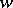which are related by an algebraic equation of special type: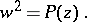(2)

Here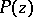is a polynomial of degree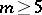without multiple roots. For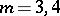one obtains elliptic integrals (cf. Elliptic integral), while the cases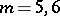are sometimes denoted as ultra-elliptic.

Equation (2) corresponds to a two-sheeted compact Riemann surfaceof genus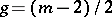ifis even, and of genusifis odd; thus, for hyper-elliptic integrals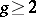. The functions,, and hence also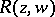, are single-valued on. The integral (1), considered as a definite integral, is given onas a curvilinear integral of an analytic function taken along some rectifiable pathand, in general, the value of the integral (1) is completely determined by a specification of the initial and final points ofalone.

As in the general case of Abelian integrals, any hyper-elliptic integral can be expressed as a linear combination of elementary functions and canonical hyper-elliptic integrals of the first, second and third kinds, having their specific forms. Thus, a normal hyper-elliptic integral of the first kind is a linear combination of hyper-elliptic integrals of the first kindwhere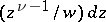,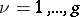, is the simplest basis of Abelian differentials (cf. Abelian differential) of the first kind for the case of a hyper-elliptic surface. Explicit expressions for Abelian differentials of the second and third kinds and for the corresponding hyper-elliptic integrals can also be readily computed . Basically, the theory of hyper-elliptic integrals coincides with the general theory of Abelian integrals.

All rational functionsof variablesandsatisfying equation (2) above form a hyper-elliptic field of algebraic functions, of genus. Any compact Riemann surface of genus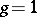or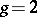has an elliptic or hyper-elliptic field, respectively. However, ifor higher, there exist compact Riemann surfacesof a complicated structure for which this assertion is no longer true.

How to Cite This Entry:
Hyper-elliptic integral. Encyclopedia of Mathematics. URL: http://encyclopediaofmath.org/index.php?title=Hyper-elliptic_integral&oldid=13571
This article was adapted from an original article by E.D. Solomentsev (originator), which appeared in Encyclopedia of Mathematics - ISBN 1402006098. See original article